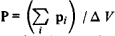# Induction, Electrical and Magnetic

The following article is from The Great Soviet Encyclopedia (1979). It might be outdated or ideologically biased.

## Induction, Electrical and Magnetic

physical quantities that, in addition to the intensities of the electric and magnetic fields, characterize an electromagnetic field. In a vacuum these characteristics coincide with the respective intensities if the CGS (Gauss) system of units is used; in the International System of Units (SI) they differ in constant factors.

Electrical induction vector. The electrical induction vector D (also called the electrical displacement) is the sum of two vectors of different nature: the electric field intensity E—the main characteristic of the field—and the polarization P, which determines the electrical state of the substance in this field. In the Gaussian system,

(1) D = E + 4πP

(4π is a constant); in the SI system

(1′) D = ε0 E + P

where ε0 is a dimensional constant, called the electric permittivity or the dielectric constant of a vacuum. The polarization vector P is an electrical dipole moment of unit volume of the substance in a field E, that is, the sum of the electrical dipole moments pi of the individual molecules within a small volume Δ V divided by the volume:In an isotropic substance that does not have ferroelectric properties, the polarization vector in weak fields is directly proportional to the field intensity. In the Gaussian system,

(3) P=keE

where кe is a dimensionless quantity called the coefficient of polarization or dielectric susceptibility. It is this factor that characterizes the substance’s electrical properties. For ferroelectrics кe depends on E, so that the relationship between P and E becomes nonlinear.

Substituting expression (3) in (1), we obtain

(4) D = (1 + 4π ke) E = єE

The quantity

(5) є = 1 + 4π ke

which also characterizes the electrical properties of the substance, is called the dielectric constant.

In the SI system,

(3’) P = keє0E

and correspondingly

(4’) D = є0єE

(5’) є = 1 + Ke

The reason for introducing the vector of electrical induction is that the flux of the vector D through any closed surface is determined only by the free charges and not by all charges within the volume bounded by the given surface, as is true for the flux of vector E. This makes it possible to avoid dealing with bound (polarized) charges and simplifies the solution of many problems.

Magnetic induction vector. The magnetic induction vector B, the main characteristic of a magnetic field, is the average value of the total intensity of the microscopic magnetic fields generated by individual electrons and other elementary particles. The vector of magnetic field intensity H is the difference of two vectors of differing nature: the vector B and the magnetization vector I. In the Gaussian system,

(6) H = B - 4πI or B= H + 4πI

The magnetization is the magnetic moment per unit volume and characterizes the magnetic state of the substance. In weak fields in an isotropic medium the magnetization is directly proportional to H:

(7) I = kmH

Where кm is the magnetic susceptibility, which characterizes the magnetic properties of the substance. For ferromagnetics кm depends on H. Substituting (7) in (6), we obtain the relation between B and H:

(8) B =(1 + 4π km) H = µH

The quantity

(9) µ = 1 + 4π km

which also characterizes the magnetic properties of a substance, is called the magnetic permeability.

In the SI system these formulas are written in the following notation:

(6′) B = µ0H + 1

(7′) I = µ0km H

(8′) B = µ0µH

(9′) µ = 1 + km

The constant μ0 is called the magnetic constant or the magnetic permeability of a vacuum. The vector H is introduced into electromagnetic field theory because of the fact that the circulation of the vector H along a closed circuit, in contrast to the circulation of the vector B, is governed only by the motion of free charges.

### REFERENCES

Kalashnikov, S. G. Elektrichestvo. Moscow, 1970. Chapters 5 and 11. (Obshchii kurs fiziki, vol. 2.)
Frish, S. E., and Timoreva, A. V. Kurs obshchei fiziki, vol. 2. Moscow, 1953. Chapters 15 and 18.

G. IA. MTAKISHEV